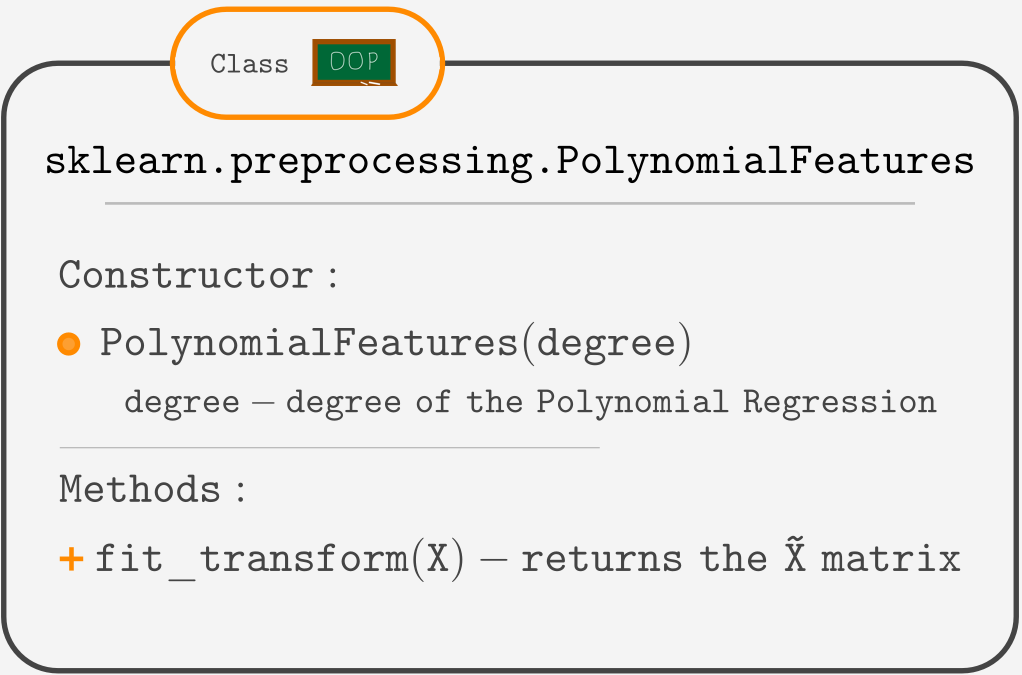Course Content

# Linear Regression with Python

Linear Regression with Python

##Building The Polynomial Regression

For this chapter, we have a file named `poly.csv`. Let's load the file and look at the contents.So here we have one feature and the target. Let's build a scatter plot.It is hard to imagine a straight line fitting this data well. So let's build a Polynomial Regression!

## Building X̃ matrix

We will once again use the `OLS` class. Still, we need to create an matrix. We do it manually by adding a squared `Feature` column to the DataFrame like this:

But if we want to build a high-degree polynomial regression, that will require adding a lot of columns like this. Luckily Scikit-Learn provides a way to do it less painfully using the `PolynomialFeatures` class.Note

`fit_transform(X)` method expects X to be either 2-d array or pandas DataFrame. If your X is an 1-d numpy array, `reshape(-1,1)` method will transform it to a 2-d array with the same contents:

If your X is a column from DataFrame, you can use `X = df[['col1']]` to get a DataFrame instead of pandas Series, which is not suited for `fit_transform()`

So to build an for the Polynomial Regression of degree `n`, we would use:

Note

The `PolynomialFeatures` class also adds a column with 1s, so you do not need to use `sm.add_constant()`.

## Building the Polynomial Regression and making the predictions

Knowing how to get an , we are ready to build the Polynomial Regression the same way as the prior models:

For predicting new values, `X_new` should be transformed using `PolynomialFeatures` too.

The following runnable example shows the entire process of building polynomial regression. `X_new` here is a 1-d array of points between -0.1 and 1.5. They are needed for visualization. And since it is a 1-d array, we should apply `reshape(-1,1)` method before using it in the PolynomialFeatures class.Feel free to play with the values of `n` in the eighth line. You will see how the plot changes depending on the polynomial regression's degree. If you pay attention, you may notice how different the predictions are for feature values lower than 0, or greater than 1.4. That is the subject of the next chapter.